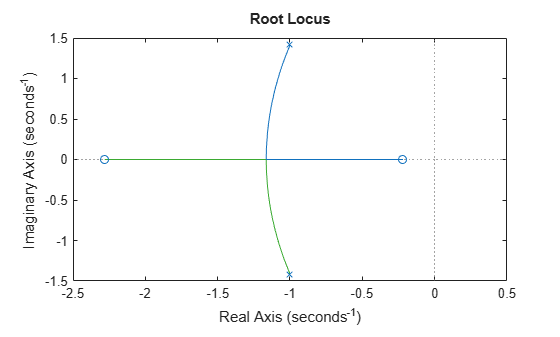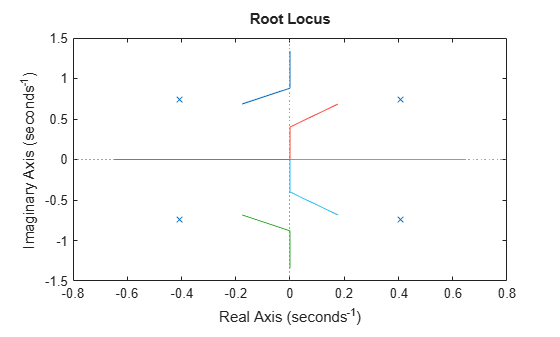rlocus

Root locus plot of dynamic system

Description

example

rlocus(sys) calculates and plots the root locus of the SISO model sys. The root locus returns the closed-loop pole trajectories as a function of the feedback gain k (assuming negative feedback). Root loci are used to study the effects of varying feedback gains on closed-loop pole locations. In turn, these locations provide indirect information on the time and frequency responses.

You can use rlocus to plot the root locus diagram of any of the following negative feedback loops by setting sys as shown below:For instance, if sys is a transfer function represented by

$sys\left(s\right)=\frac{n\left(s\right)}{d\left(s\right)}$

the closed-loop poles are the roots of

$d\left(s\right)+kn\left(s\right)=0$

The root locus plot depicts the trajectories of closed-loop poles when the feedback-gain k varies from 0 to infinity. rlocus adaptively selects a set of positive gains k to produce a smooth plot. The poles on the root locus plot are denoted by x and the zeros are denoted by o.

example

rlocus(sys1,sys2,...) plots the root loci of multiple LTI models sys1, sys2,... on a single plot. You can specify a color, line style, and marker for each model. For even more plot customization options, see rlocusplot.

example

[r,k] = rlocus(sys) returns the vector of feedback gains k and the complex root locations r for these gains.

example

r = rlocus(sys,k) uses the user-specified vector of feedback gains k to output the closed-loop poles r that define the root locus plot.

Examples

collapse all

For this example. plot the root-locus of the following SISO dynamic system:

$sys\left(s\right)=\frac{2{s}^{2}+5s+1}{{s}^{2}+2s+3}.$

sys = tf([2 5 1],[1 2 3]);
rlocus(sys)The poles of the system are denoted by x, while the zeros are denoted by o on the root locus plot. You can use the menu within the generated root locus plot to add grid lines, zoom in or out, and also invoke the Property Editor to customize the plot.

For more plot customization options, use rlocusplot.

For this example, consider sisoModels.mat which contains the following three SISO models:

• sys1 $-$ a transfer function model

• sys2 $-$ a state-space model

• sys3 $-$ a zero-pole-gain model

Load the models from the mat file.

Create the root locus plot using rlocus and specify the color for each system. Also add a legend to the root locus plot.

rlocus(sys1,'b',sys2,'k',sys3,'r')
hold on
legend('sys1','sys2','sys3')
hold offThe figure contains root locus diagrams for all three systems in the same plot. For more plot customization, see rlocusplot.

For this example, consider the following SISO transfer function model:

$sys\left(s\right)=\frac{3{s}^{2}+1}{9{s}^{3}+7{s}^{2}+5s+6}$

Use the above transfer function model with rlocus to extract the closed-loop poles and associated feedback gain values.

sys = tf([3 1],[9 7 5 6]);
[r,k] = rlocus(sys)
r = 3×53 complex

-0.9406 + 0.0000i  -0.8744 + 0.0000i  -0.8685 + 0.0000i  -0.8620 + 0.0000i  -0.8550 + 0.0000i  -0.8475 + 0.0000i  -0.8394 + 0.0000i  -0.8306 + 0.0000i  -0.8212 + 0.0000i  -0.8111 + 0.0000i  -0.8003 + 0.0000i  -0.7888 + 0.0000i  -0.7766 + 0.0000i  -0.7636 + 0.0000i  -0.7500 + 0.0000i  -0.7358 + 0.0000i  -0.7209 + 0.0000i  -0.7055 + 0.0000i  -0.6896 + 0.0000i  -0.6734 + 0.0000i  -0.6569 + 0.0000i  -0.6402 + 0.0000i  -0.6236 + 0.0000i  -0.6071 + 0.0000i  -0.5908 + 0.0000i  -0.5748 + 0.0000i  -0.5593 + 0.0000i  -0.5443 + 0.0000i  -0.5299 + 0.0000i  -0.5161 + 0.0000i  -0.5030 + 0.0000i  -0.4906 + 0.0000i  -0.4789 + 0.0000i  -0.4679 + 0.0000i  -0.4576 + 0.0000i  -0.4480 + 0.0000i  -0.4390 + 0.0000i  -0.4306 + 0.0000i  -0.4229 + 0.0000i  -0.4157 + 0.0000i  -0.4090 + 0.0000i  -0.4029 + 0.0000i  -0.3972 + 0.0000i  -0.3919 + 0.0000i  -0.3871 + 0.0000i  -0.3826 + 0.0000i  -0.3785 + 0.0000i  -0.3748 + 0.0000i  -0.3713 + 0.0000i  -0.3681 + 0.0000i
0.0814 + 0.8379i   0.0483 + 0.9140i   0.0453 + 0.9212i   0.0421 + 0.9291i   0.0386 + 0.9377i   0.0349 + 0.9470i   0.0308 + 0.9573i   0.0264 + 0.9686i   0.0217 + 0.9809i   0.0167 + 0.9943i   0.0113 + 1.0090i   0.0055 + 1.0251i  -0.0006 + 1.0426i  -0.0071 + 1.0617i  -0.0139 + 1.0826i  -0.0210 + 1.1053i  -0.0284 + 1.1300i  -0.0362 + 1.1568i  -0.0441 + 1.1859i  -0.0522 + 1.2175i  -0.0605 + 1.2515i  -0.0688 + 1.2883i  -0.0771 + 1.3278i  -0.0853 + 1.3703i  -0.0935 + 1.4158i  -0.1015 + 1.4644i  -0.1092 + 1.5162i  -0.1167 + 1.5714i  -0.1239 + 1.6299i  -0.1308 + 1.6920i  -0.1374 + 1.7578i  -0.1436 + 1.8273i  -0.1494 + 1.9006i  -0.1549 + 1.9780i  -0.1601 + 2.0594i  -0.1649 + 2.1452i  -0.1694 + 2.2354i  -0.1736 + 2.3302i  -0.1775 + 2.4299i  -0.1810 + 2.5345i  -0.1844 + 2.6442i  -0.1875 + 2.7594i  -0.1903 + 2.8802i  -0.1929 + 3.0069i  -0.1953 + 3.1397i  -0.1976 + 3.2789i  -0.1996 + 3.4247i  -0.2015 + 3.5775i  -0.2032 + 3.7375i  -0.2048 + 3.9052i
0.0814 - 0.8379i   0.0483 - 0.9140i   0.0453 - 0.9212i   0.0421 - 0.9291i   0.0386 - 0.9377i   0.0349 - 0.9470i   0.0308 - 0.9573i   0.0264 - 0.9686i   0.0217 - 0.9809i   0.0167 - 0.9943i   0.0113 - 1.0090i   0.0055 - 1.0251i  -0.0006 - 1.0426i  -0.0071 - 1.0617i  -0.0139 - 1.0826i  -0.0210 - 1.1053i  -0.0284 - 1.1300i  -0.0362 - 1.1568i  -0.0441 - 1.1859i  -0.0522 - 1.2175i  -0.0605 - 1.2515i  -0.0688 - 1.2883i  -0.0771 - 1.3278i  -0.0853 - 1.3703i  -0.0935 - 1.4158i  -0.1015 - 1.4644i  -0.1092 - 1.5162i  -0.1167 - 1.5714i  -0.1239 - 1.6299i  -0.1308 - 1.6920i  -0.1374 - 1.7578i  -0.1436 - 1.8273i  -0.1494 - 1.9006i  -0.1549 - 1.9780i  -0.1601 - 2.0594i  -0.1649 - 2.1452i  -0.1694 - 2.2354i  -0.1736 - 2.3302i  -0.1775 - 2.4299i  -0.1810 - 2.5345i  -0.1844 - 2.6442i  -0.1875 - 2.7594i  -0.1903 - 2.8802i  -0.1929 - 3.0069i  -0.1953 - 3.1397i  -0.1976 - 3.2789i  -0.1996 - 3.4247i  -0.2015 - 3.5775i  -0.2032 - 3.7375i  -0.2048 - 3.9052i

k = 1×53
104 ×

0    0.0001    0.0001    0.0001    0.0001    0.0001    0.0001    0.0001    0.0001    0.0001    0.0001    0.0001    0.0002    0.0002    0.0002    0.0002    0.0002    0.0003    0.0003    0.0003    0.0003    0.0004    0.0004    0.0004    0.0005    0.0005    0.0006    0.0006    0.0007    0.0007    0.0008    0.0009    0.0010    0.0011    0.0012    0.0013    0.0014    0.0015    0.0017    0.0018    0.0020    0.0022    0.0024    0.0026    0.0028    0.0031    0.0034    0.0037    0.0041    0.0045

Since sys contains 3 poles, the size of the resultant array of poles r is 3x53. Each column in r corresponds to a gain value from vector k. For this example, rlocus automatically chose 53 values of k from zero to infinity to obtain a smooth trajectory for the three closed-loop poles.

display(r(:,39))
-0.4229 + 0.0000i
-0.1775 + 2.4299i
-0.1775 - 2.4299i
display(k(39))
16.5907

For instance, r(:,39) contains the above closed-loop poles for a feedback gain value of 16.5907.

For this example, consider the following SISO transfer function model:

$sys\left(s\right)=\frac{0.5{s}^{2}-1}{4{s}^{4}+3{s}^{2}+2}$

Define the transfer function model and required vector of feedback gain values. For this example, consider a set of gain values varying from 1 to 8 with increments of 0.5 and extract the closed-loop pole locations using rlocus.

sys = tf([0.5 -1],[4 0 3 0 2]);
k = (1:0.5:5);
r = rlocus(sys,k);
size(r)
ans = 1×2

4     9

Since sys contains 4 closed-loop poles, the size of the resultant array of closed-pole locations r is 4x9 where the 9 columns correspond to the 9 specific gain values defined in k.

You can also visualize the trajectory of the closed-loop poles for the specific gain values in k on the root locus plot.

rlocus(sys,k)Input Arguments

collapse all

SISO dynamic system, specified as one of the following:

• Continuous-time or discrete-time numeric LTI models which include tf, zpk, or ss models.

• Generalized or uncertain LTI models such as genss or uss (Robust Control Toolbox) models. (Using uncertain models requires Robust Control Toolbox™ software.)

rlocus assumes

• current values of the tunable components for tunable control design blocks.

• nominal model values for uncertain control design blocks.

• Identified LTI models, such as idtf (System Identification Toolbox), idss (System Identification Toolbox), idproc (System Identification Toolbox), idpoly (System Identification Toolbox), and idgrey (System Identification Toolbox) models. (Using identified models requires System Identification Toolbox™ software.)

Feedback gain values that pertain to pole locations, specified as a vector. The feedback gains define the trajectory of the poles thereby affecting the shape of the root locus plot.

Output Arguments

collapse all

Closed-loop pole locations of sys corresponding to each value in k, returned as an n-by-m array, where n is the number of closed-loop poles of sys and m = max(length(k)).

Feedback gain values that pertain to pole locations, returned as a vector. The feedback gains define the trajectory of the poles thereby affecting the shape of the root locus plot. When k is not defined by the user, rlocus adaptively selects a set of positive gains k between zero and infinity, to produce a smooth plot.